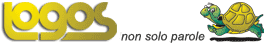Select LanguageIsaac Newton (1642-1727)   English physicist and mathematician who laid the foundations of physics as a modern discipline. During 166566, he discovered the binomial theorem, differential and integral calculus, and that white light is composed of many colours. He developed the three standard laws of motion (see Newton's laws of motion) and the universal law of gravitation, set out in Philosophiae naturalis principia mathematica (1687), usually referred to as the Principia. He was knighted in 1705.Newton's greatest achievement was to demonstrate that scientific principles are of universal application. He clearly defined the nature of mass, weight, force, inertia, and acceleration.In 1679 Newton calculated the Moon's motion on the basis of his theory of gravity and also found that his theory explained the laws of planetary motion that had been derived by German astronomer Johannes Kepler on the basis of observations of the planets.